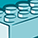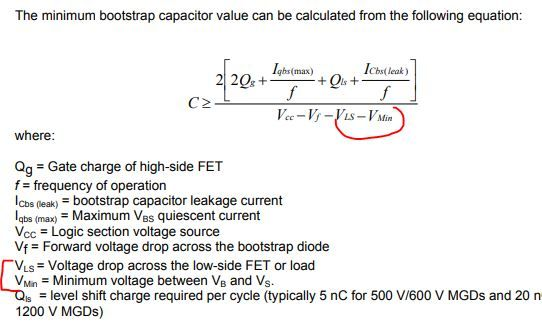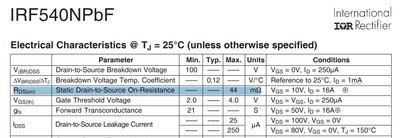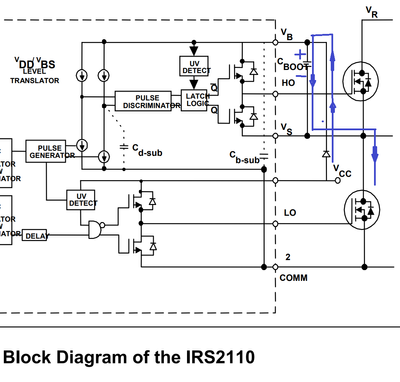Announcements

# MOSFET (Si/SiC) Forum DiscussionsLevel 1
Level 1# unknowns of values to find the bootstrap capacitor

Hello, I am implementing the following formula of the "Application Note AN-978", by chance someone can guide me about the value of Vls and Vmin, I do not know in which part of the datasheet this or what value to put, I would appreciate it if you can help me thanks. I am using the IR2110 controller and the IRF540N mosfet.

https://www.infineon.com/dgdl/Infineon-HV_Floating_MOS_Gate_Drivers-ApplicationNotes-v01_00-EN.pdf?f...1 SolutionModeratorModerator# Re: unknowns of values to find the bootstrap capacitor

Hi @WilliamC,

The mentioned Vmin value (7.5V) is low in your case which may turn off the MOSFET, since it is below the Vbs (Under Voltage) value. Ref datasheet-

https://www.infineon.com/dgdl/Infineon-IR2110-DataSheet-v01_00-EN.pdf?fileId=5546d462533600a4015355c...

Also, the Vls value is the ON state voltage drop across your MOSFET, i.e.,

Vls= Rds(ON)*Ids (ON); Note that Ids (ON)= (Load current+ Iqbs max)

Consider Rds (ON) as given below-In general, this bootstrap capacitor should be at least 10 times greater than the
gate capacitance of the high-side MOSFET. Because it should have enough energy to charge the gate of the high-side MOSFET without getting discharged by more than 10%.

BR

Shivam

5 RepliesModeratorModerator# Re: unknowns of values to find the bootstrap capacitor

Dear @WilliamC

During the selection of Bootstrap capacitance value,  Vls and Vmin values are required to calculate the net voltage across the capacitor while it is getting charged. The functioning of this capacitance involves charging and discharging modes, where charging mode voltage is required for capacitance calculation. During the charging mode the path of current will be as shown below-While the capacitor is getting charged, the net voltage is across the capacitor will be-

(Vcc-Vf-Vmin-Vls)

The load current is required to calculate the Vls for the MOSFET. Since, Rds ON differs for different MOSFETs.

Also, the voltage across the capacitor contains ripples, which corresponds to some finite Vmax and Vmin.

You can calculate the capacitance values by observing these values and the values given in datasheet.

However, if you still face any calculation challenge, pl let me know the below mentioned values for further calculations-

• Part number of both High side and Low side MOSFET, if both the FETs are different.
• Voltage ripples across the capacitance and Vgs value for your application.
• Type of converter topology.
• Value of maximum load current.

Kind Regards

ShivamLevel 1
Level 1# Re: unknowns of values to find the bootstrap capacitor

Dear @ShivamPandey

I appreciate the information and help provided, it is of great help to me to understand these incognitas in theoretical terms if I understand you and thanks to that I could already get Vmin, however it has been difficult for me to find "VLS", I would appreciate your guidance either through an example with numbers or something like that, thank you very much.
I am currently implementing the full bridge topology, the components that I am implementing are the IR2110 driver and the IRF540N mosfet, I share the datasheet
https://www.infineon.com/dgdl/Infineon-IR2110-DataSheet-v01_00-EN.pdf?fileId=5546d462533600a4015355c...

https://www.infineon.com/dgdl/Infineon-IRF540N-DataSheet-v01_01-EN.pdf?fileId=5546d462533600a4015355...

These are some data I have
Qg=72nC
Iqbs=230uA
Frequency=25000 Hz
Qls=5nC
ICbs= in this case I am not taking it into account since I am not using the electrolytic type.
Vdc= 15v
Vf=0.7
Vls= ¿?
Vmin=7.5
I still don't know how to interpret what you are telling me or how to analyze the graph to obtain the value of "VLS".

to be honest this is the incognita that I have not been able to know how to get it, everyone says that the data said but I still do not know, although I have read and looked at many documents and guides, I still can not 😞ModeratorModerator# Re: unknowns of values to find the bootstrap capacitor

Hi @WilliamC,

The mentioned Vmin value (7.5V) is low in your case which may turn off the MOSFET, since it is below the Vbs (Under Voltage) value. Ref datasheet-

https://www.infineon.com/dgdl/Infineon-IR2110-DataSheet-v01_00-EN.pdf?fileId=5546d462533600a4015355c...

Also, the Vls value is the ON state voltage drop across your MOSFET, i.e.,

Vls= Rds(ON)*Ids (ON); Note that Ids (ON)= (Load current+ Iqbs max)

Consider Rds (ON) as given below-In general, this bootstrap capacitor should be at least 10 times greater than the
gate capacitance of the high-side MOSFET. Because it should have enough energy to charge the gate of the high-side MOSFET without getting discharged by more than 10%.

BR

ShivamLevel 1
Level 1# Re: unknowns of values to find the bootstrap capacitor

Dear @ShivamPandey

ready, I understand you my Vmin I have decided to change it to 9.5 taking into account the datasheet of the components and taking into account your advice, sorry to bother you so much with this topic. As for Ids (ON) = (Load current + Iqbs max), I know that Iqbs is in the datasheet, I know what the value is, but the load current would be the one that more or less my whole system would use, or it would be the current of each mosfet? (I use 4 of the IRF540N) and two IR2110 driver.

Kind Regards

WilliamCModeratorModerator# Re: unknowns of values to find the bootstrap capacitor